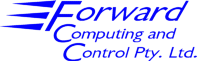Home | pfodApps/pfodDevices | WebStringTemplates | Java/J2EE | Unix | Torches | Superannuation | Contact | About UsSimGauss™ V2.0 Digitally Controlled Spring SystemEquations

M x dv/dt = -M x g + K x (x0 - x) - R x v
dx/dt = v

Every 0.1 seconds the velocity is sampled by the digital
controller and Rd calculated from
Rd = R0 + abs(v) x gain

This calculation takes 10ms which is modelled by a delay
before the new value of damping is transferred to the
system damping parameter, R, via
R = Rd

where:-
K     is the spring constant (Kg/s2)
M     the mass (Kg)
R     the damping coefficient (Kg/s)
Rd    the damping coefficient calculated by the controller
R0    the minimum system damping coefficient
gain  the gain of the digital controller
x0    the length of the spring at rest (m)
g     the gravitational acceleration constant (m/s2)
v     the velocity of the mass (m/s)
x     the position of the mass (m)

Contact Forward Computing and Control by
©Copyright 1996-2017 Forward Computing and Control Pty. Ltd. ACN 003 669 994